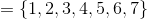## Example Questions

### Example Question #8 : Venn Diagrams

Given the Venn diagram below, which of the following does not belong to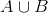?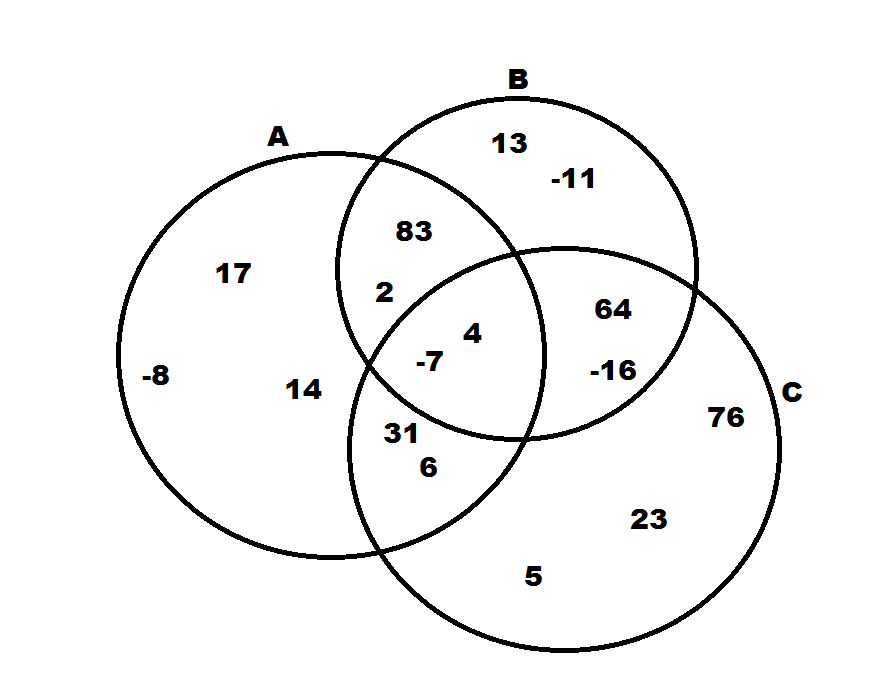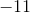Explanation:

The symbol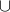stands for the union between two sets.  Therefore,means the set of all numbers that are in either A or B.  Looking at our choices, the only number that isn't in either A, B, or both is 23.

### Example Question #9 : Venn Diagrams

Sixty high school seniors were polled to see if they were taking history and calculus. A total of 29 students said they were taking calculus, and a total of 50 students said they were taking history. What is the minimum number of students who take both history and calculus?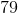Explanation:

We can draw a Venn diagram to see these two sets of students.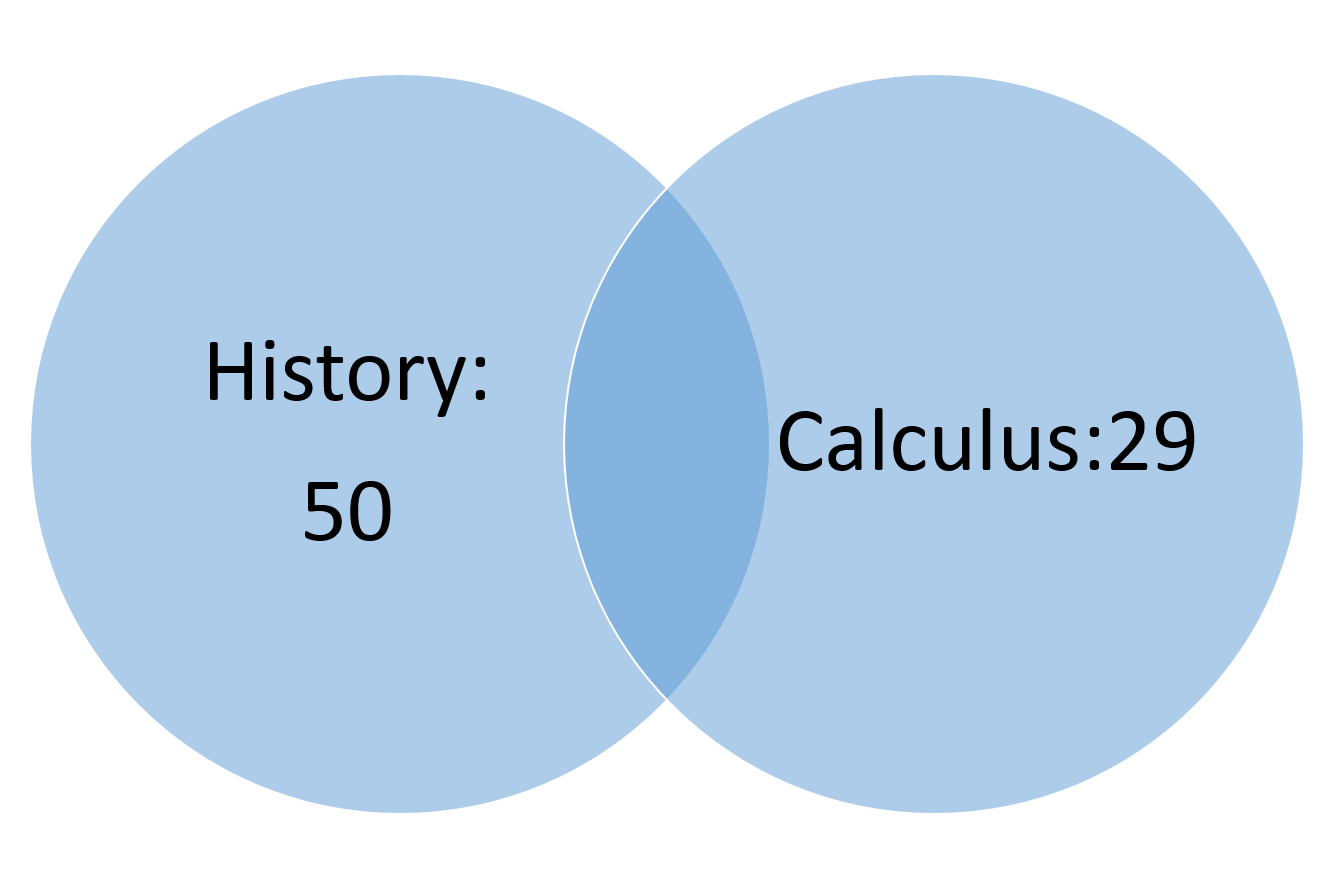We need to find the overlap between these two sets. To find that, add up the total number of students who are taking history and the total number of students who are taking calculus.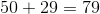Notice that we have more students this way than the total number who were polled. That is because the students who are taking history AND calculus have been double counted. Subtract the total number of students polled to find out how many students were counted twice.

### Example Question #10 : Venn Diagrams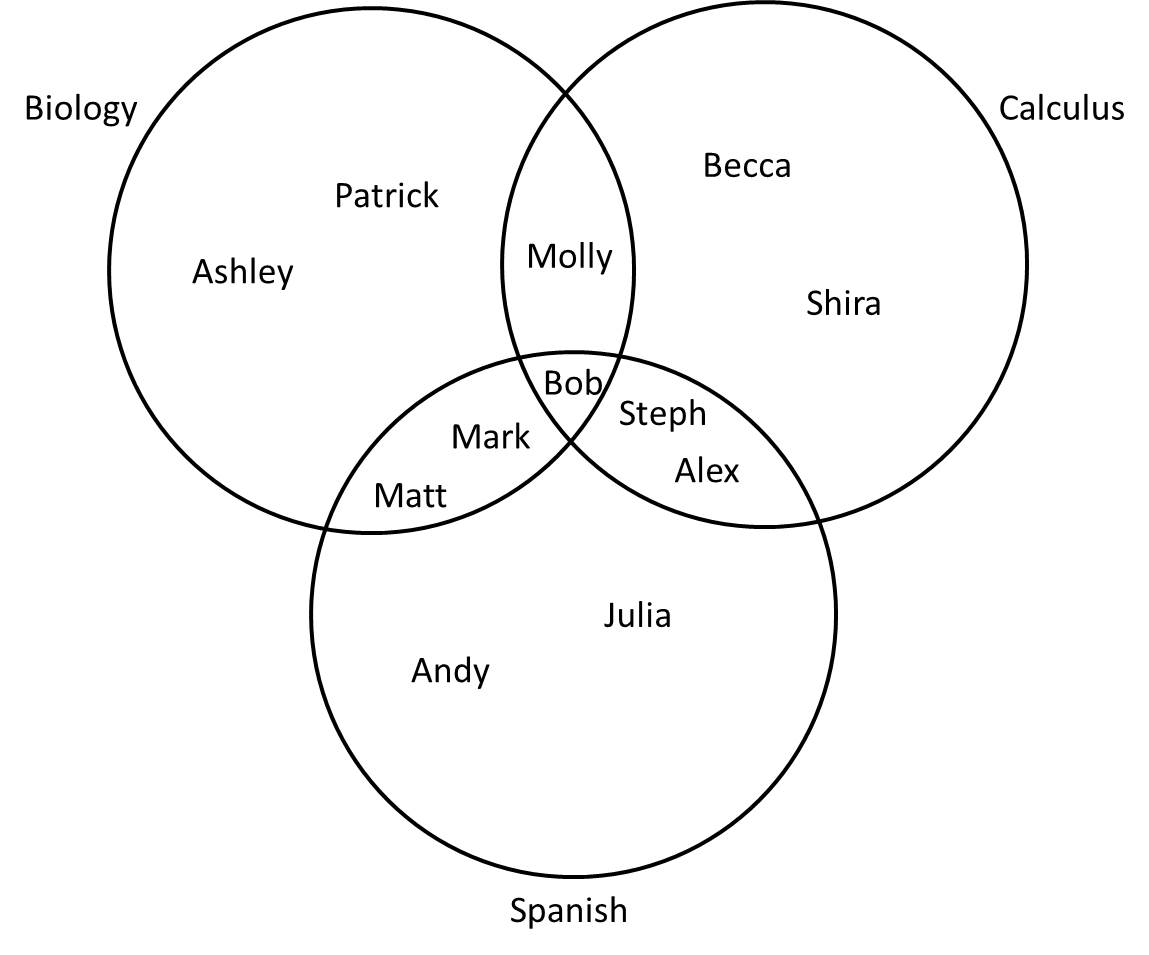A group of high school juniors are taking Biology, Calculus, and Spanish as shown above. Which student is not in the set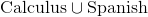?

Bob

Patrick

Steph

Andy

Molly

Patrick

Explanation:

The notationstands for "union," which refers to everything that is in either set.refers to the group of students taking either Calculus or Spanish (everyone on this diagram except those taking only Biology). From the diagram, Patrick and Ashley are the only students taking neither Calculus nor Spanish, so Patrick is the correct answer.

### Example Question #1 : How To Find The Union Of A Venn Diagram

Forty students play soccer and/or basketball after school. Twenty-four students play soccer and twenty-nine play basketball. How many students play both soccer and basketball?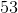Explanation:

We can draw a Venn diagram of these students.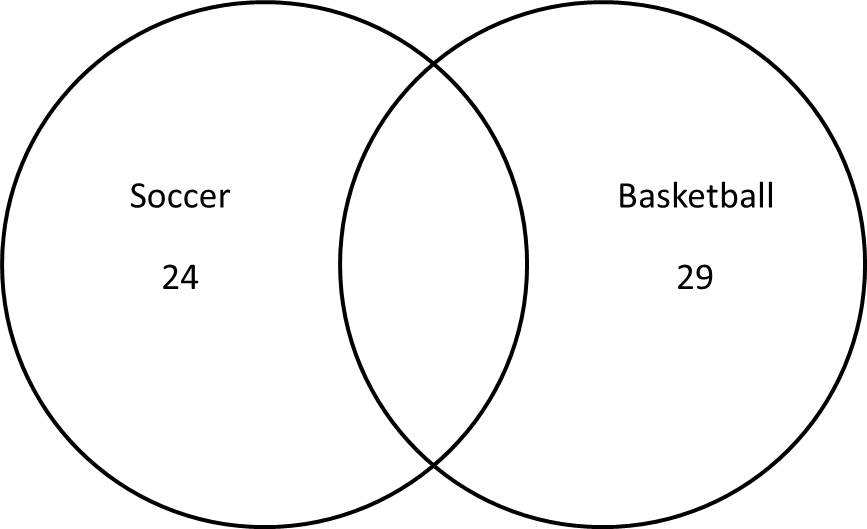Drawn this way, there are more students on the Venn diagram than we have.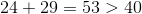This is because some of the students play both sports and should be in the overlap on the Venn diagram. To find the number of students in the overlap, subtract the total number of students given from the number on the diagram.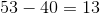This represents the number of students who were counted twice, or the number in the overlap.

We can redraw the correct Venn diagram with this number.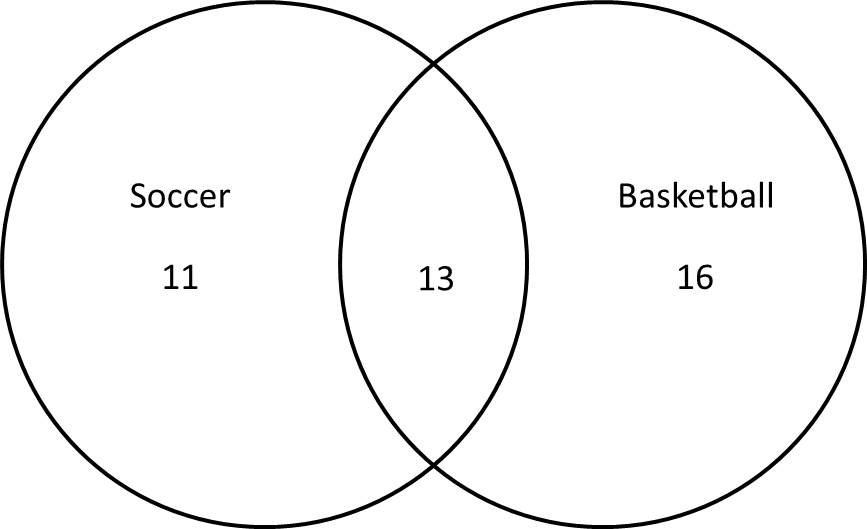### Example Question #2 : How To Find The Union Of A Venn Diagram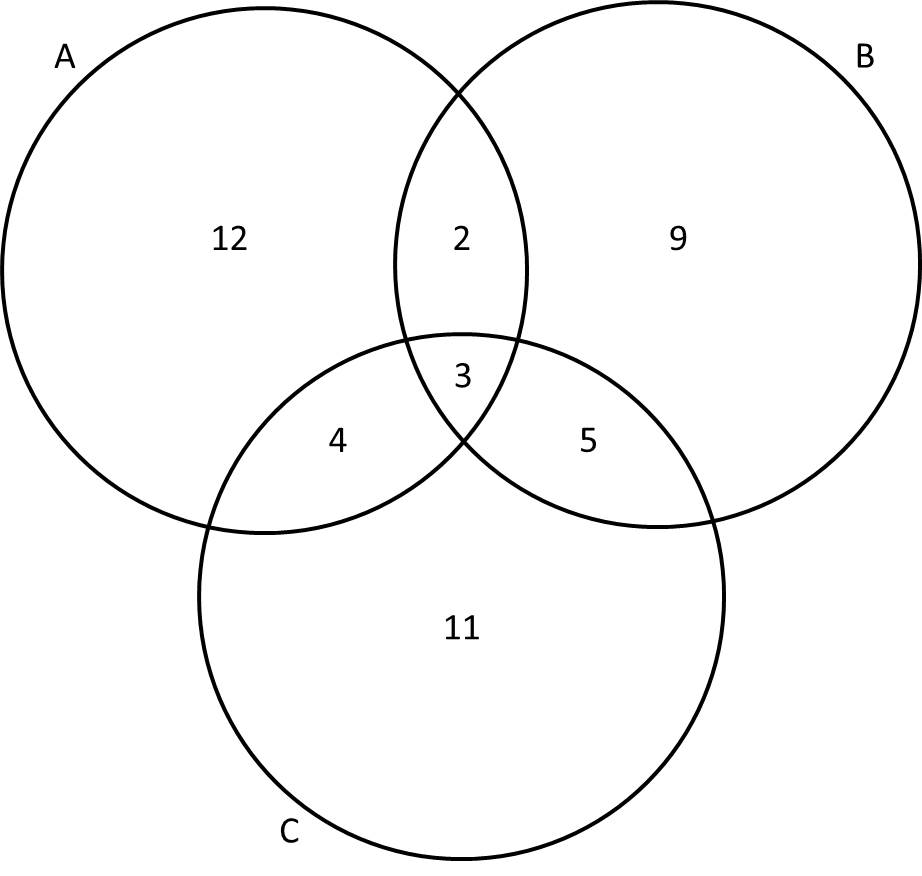Giving the Venn diagram above, what is the sum of the numbers in the set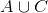?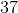Explanation:

The notationstands for "A union C," which refers to everything that is in either setor set.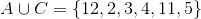When we add the numbers together, we get: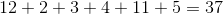### Example Question #3 : How To Find The Union Of A Venn Diagram

In a class of senior high-school students,have pet cats,have pet dogs,have both cats and dogs, andhave neither cats nor dogs. How many total students are in the class?Explanation:

A Venn diagram can help us determine the total number of students in the class.

First, we must calculate the number of students who have ONLY cats or ONLY dogs. First, for cats, 15 students have cats, and 5 students have both cats and dogs.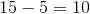Ten students have only cats.

For dogs, 12 students have dogs, and 5 students have both cats and dogs.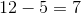Seven students have only dogs.

Using this information, we can fill in the Venn diagram.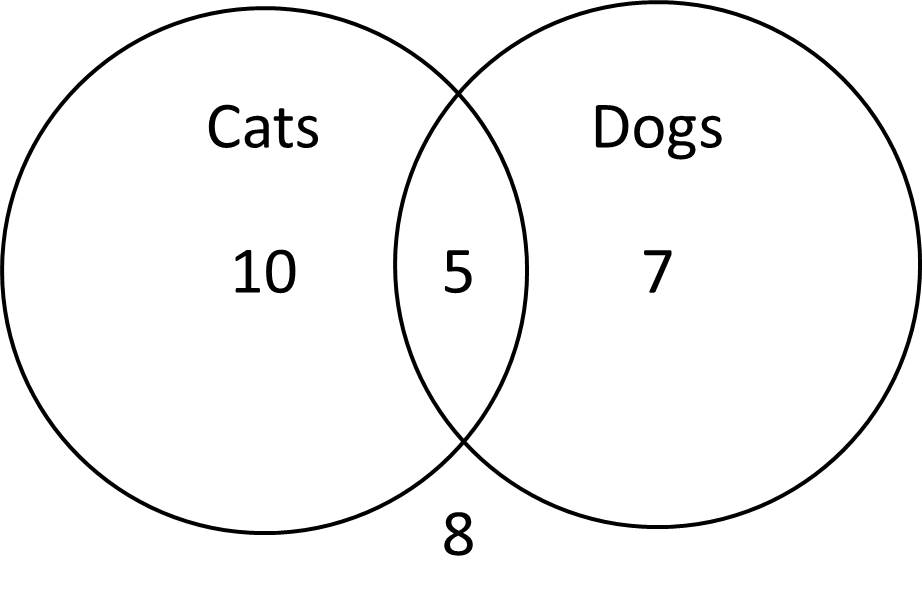This diagram shows the 10 students with only cats, the 7 students with only dogs, the 5 students with both, and the 8 students with neither.  Adding up the numbers will give us the total number of students.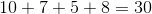### Example Question #4 : How To Find The Union Of A Venn Diagram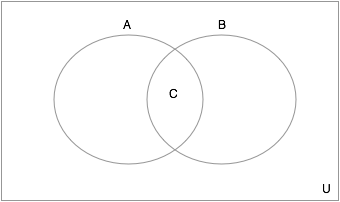In the venn diagram above, let the set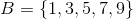and let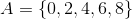, what is the set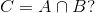Use set notation to enumerate your answer.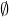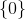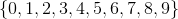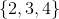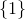Explanation: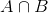means the intersection of the setsand, the only things in the intersection are elementes that are in BOTH of the sets. Since there is nothing shared by the two sets (no elements are in both), the interesction is the emptyset, or:### Example Question #5 : How To Find The Union Of A Venn Diagram

In a venn diagram, let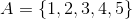and let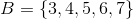What is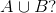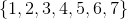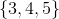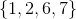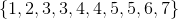is the union of the setsandwhich is the set that contains anything that is in either set. Thus, the total ofis every element that is in one of the two sets, and so